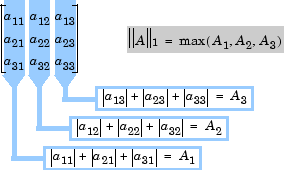# Reciprocal Condition

Compute reciprocal condition of square matrix in 1-norm

## Library

Math Functions / Matrices and Linear Algebra / Matrix Operations

`dspmtrx3`

•## Description

The Reciprocal Condition block computes the reciprocal of the condition number for a square input matrix A.

```y = rcond(A) % Equivalent MATLAB code ```

or

`$y=\frac{1}{\kappa }=\frac{1}{{‖{A}^{-1}‖}_{1}{‖{A}^{}‖}_{1}}$`

where κ is the condition number (κ ≥ 1), and y is the scalar output (0 ≤ y < 1).

The matrix 1-norm, ${‖A‖}_{1}$, is the maximum column-sum in the M-by-M matrix A.

`${‖A‖}_{1}=\stackrel{\mathrm{max}}{1\le j\le M}\sum _{i=1}^{M}|{a}_{ij}|$`

For a 3-by-3 matrix:## References

Golub, G. H., and C. F. Van Loan. Matrix Computations. 3rd ed. Baltimore, MD: Johns Hopkins University Press, 1996.

## Supported Data Types

• Double-precision floating point

• Single-precision floating point

 Matrix 1-Norm DSP System Toolbox Normalization DSP System Toolbox `rcond` MATLAB